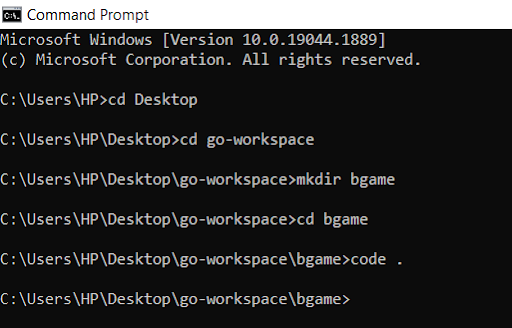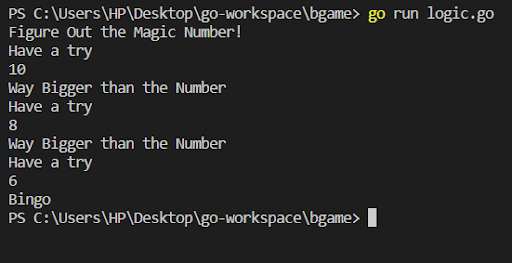Beginner
Intermediate
Blockchain
Comparison

# How to Develop Golang Games? - Guide for BeginnersDeveloping games in Golang, huh? Wow, that’s a big step! But if you are a beginner or an intermediate, do not be disheartened. You can always start small and move on to bigger projects if you have a grasp of the fundamental concepts. In this blog, we will take a look at Golang game programming and utilize it to create a simple game- Judging What the Random Number might be.

Are you ready?? Great! Let’s get started.

Prerequisites

You need to have the Go compiler installed and an IDE (for this tutorial, we will be using VS Code).

## Create a New Directory

• I would like to start by creating a new directory. For this, we will need to open Command Prompt in windows or terminal in Mac or linux . Usually, I store all the projects inside go-workspace located on the Desktop. And that’s what we will do.• As you can see, we have created a directory named ‘bgame’ and we have opened VS Code. So, now let’s get to the fun part.

## Coding in VS Code

• We create a file named ‘logic.go’ inside the directory ‘bgame’. You will get to see that in Golang, the packages are responsible for controlling the source files. Now, we start typing the code.
```1 2 3 4 5 6 7 ``````package main import ( “fmt” “time” “math/rand” ) ```
• Here, we are importing three packages.

fmt- It helps in implementing the formatted input and output. It also helps you to format values, basic strings, etc. and print them.

time- Golang has good support for durations and times. And it is provided by the package time.

math/rand- Pseudorandom number generators are implemented by package rand, which is not suited for operations involving sensitive security.

• Now, we move on with the rest of the program.
```1 2 ``````var try int var testtally int= 1 ```
• Here, we declare two variables, both of type integer. The ‘try’ variable helps in storing the guess when the user is asked to input. The ‘testtally’ records the number of times the compiler has been the recipient of a ‘try’. Now, we will write the main function.
```1 2 3 4 ``````func main() { origin := rand.NewSource(time.Now().UnixNano()) random := rand.New(origin) confidential := random.Intn (10) ```
• Ok, so let us understand what we have written in this part of Golang game programming.

-We have started writing the main function. As that is a prerequisite to start programming. Go programming begins with func main execution. Since we want to generate unique variables, every time we tell the code to run, we get back time, which is always unique. This is the function of the ‘origin’. Thus, the ‘random’ takes a timestamp and stores it in the ‘origin’.

-Now, the ‘random’ takes the ‘origin’ and it initializes it as the exact timestamp. The ‘random’ variable actually asks the compiler to choose any random number at an instantaneous time than what has been satiated in the ‘origin’.

-The ‘confidential’ variable lets the ‘random’ variable know that the random number should fall within the range of 0 to 10. Furthermore, the ‘confidential’ variable should also store the number.

• Moving on with the code
```1 2 3 4 5 6 7 8 9 10 11 12 13 14 15 16 17 18 19 20 21 22 23 ``````fmt.Println("Figure Out the Magic Number!") for { fmt.Println ("Have a try") fmt.Scan (&try) if testtally > 5 { fmt.Println ("Bad Luck") break } else { if try > confidential { fmt.Println ("Way Bigger than the Number") } else if try < confidential { fmt.Println ("Way Smaller than the Number") } else { fmt.Println ("Bingo") break } } testtally ++ } } ```

And with this the Golang game programming has come to an end. But we have a lot to catch up on. I’ll explain the program covering all the concepts.

• With the fmt.Println(“Figure Out the Magic Number!”

I’m asking the compiler to print out the exact line.

Following this, we create a for loop, within which I have included a set of instructions.

• The fmt.Println (“Have a Try”) is asking the compiler to ask for an integer from the user (player). It then scans the input with fmt.Scan(&try), and the input is passed into variable ‘try’. The ampersand sign is a pointer and it tells the variable to put the input in ‘try’
• Then we ask the compiler to check if the testtally is greater than 5, in which case it displays the message “You Lose”
• If the condition is not satisfied, then we move on to ‘else’. If the ‘try’ variable is more than the ‘confidential’ number, we print that “Way Bigger than the Number”
• If this particular condition is not satisfied, we move on to ‘else if’. If the ‘try’ is smaller than the ‘confidential’ number, then we print “Way Smaller than the Number”
• Other than these options, the only option left is the chance of the player winning the game. So, we create a condition with else again where we print “Bingo”.
• Finally, the testtally ++ statement signifies that we increase the trial one after the other. Now, all that is left is to try and run the program.

## Run the Code in the Terminal

Now, you have to run the code in the terminal. For that you type:

`go run logic.go`

As soon as you do so, the compiler will showcase

“Figure Out the Magic Number!”

“Have a Try”As I type 10, it tells me that the ‘confidential’ number is smaller than my ‘try’. So, I go at it again. This time, I type 8, still it lets me know that the number is smaller than the ‘try’.

Finally, I type 6 and it works. I get a message “Bingo” just as I have typed in the program.

Hopefully, you have understood the program. Good! You can create similar basic games just by applying logic. The more you code, you will be able to develop your programming prowess. Eventually, this will help you out in creating big projects, where you have more than one source file to think about.

You can find the code from https://github.com/GolangCompany/basic-game-